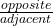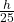## At a point 25 ft. from the base of a totem pole, the angle of elevation of the top of the pole is 50.1 °. How tall is the totem pole to the

Question

At a point 25 ft. from the base of a totem pole, the angle of elevation of the top of the pole is 50.1 °. How tall is the totem pole to the nearest foot?

in progress 0
6 months 2021-07-30T15:05:22+00:00 1 Answers 13 views 0

## Answers ( )

height ≈ 30 ft

Step-by-step explanation:

The situation is modelled by a right triangle.

let h be the height of the totem pole, then

tan50.1° ==( multiply both sides by 25 )

25 × tan50.1° = h , then

h ≈ 30 ft ( to the nearest foot )